ACT  >  Integers

# Integers - Notes | Study Mathematics for ACT - ACT

 Table of contentsIntroduction1 Crore+ students have signed up on EduRev. Have you?

Introduction

Integers include positive numbers, negative numbers, and zero. 'Integer' is a Latin word which means 'whole' or 'intact'. This means integers do not include fractions or decimals.

What is an Integer?

Integers include all whole numbers and negative numbers. This means if we include negative numbers along with whole numbers, we form a set of integers.

Definition of Integers

An integer is a number with no decimal or fractional part and it includes negative and positive numbers, including zero. A few examples of integers are: -5, 0, 1, 5, 8, 97, and 3,043. A set of integers, which is represented as Z, includes:

• Positive Numbers: A number is positive if it is greater than zero. Example: 1, 2, 3 . . .
• Negative Numbers: A number is negative if it is less than zero. Example: -1, -2, -3 . . .
• Zero is defined as neither a negative number nor a positive number. It is a whole number.

Z = {... -7, -6, -5, -4, -3, -2, -1, 0, 1, 2, 3, ...}
Observe the figure given below to understand the definition of integers.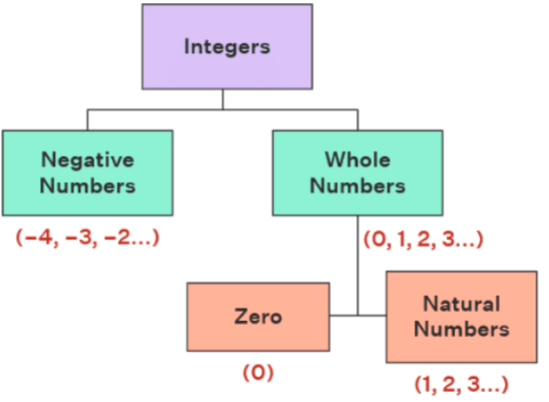Integers on a Number Line

A number line is a visual representation of numbers on a straight line. This line is used for the comparison of numbers that are placed at equal intervals on an infinite line that extends on both sides, horizontally. Just like other numbers, the set of integers can also be represented on a number line.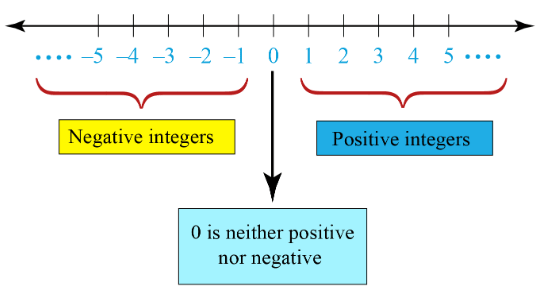Graphing Integers on a Number Line

Positive and negative integers can be visually represented on a number line. Integers on a number line help in performing arithmetic operations. The basic points to keep in mind while placing integers on a number line are as follows:

• The number on the right side is always greater than the number on the left side.
• Positive numbers are placed on the right side of 0, because they are greater than 0.
• Negative numbers are placed on the left side of 0, because they are smaller than 0.
• Zero, which is not positive or negative, is usually kept in the middle.

Integer Operations

The four basic arithmetic operations associated with integers are:

• Subtraction of Integers
• Multiplication of Integers
• Division of Integers

There are some rules for performing these operations of integers. Before we start learning these methods of integer operations, we need to remember a few things.

• If there is no sign in front of a number, it means that the number is positive. For example, 5 means +5.
• The absolute value of an integer is a positive number, i.e., |−2| = 2 and |2| = 2.

Adding integers is the process of finding the sum of two or more integers where the value might increase or decrease depending on the integer being positive or negative. The different rules and the possible cases for the addition of integers are given in the following section.

While adding two integers, we use the following rules:

• When both integers have the same signs: Add the absolute values of integers, and give the same sign as that of the given integers to the result.
• When one integer is positive and the other is negative: Find the difference of the absolute values of the numbers and then give the sign of the larger of these numbers to the result.

Example 1: Add the given integers: 2 + (-5)
Solution:
Here, the absolute values of 2 and (-5) are 2 and 5 respectively.
Their difference (larger number - smaller number) is 5 - 2 = 3
Now, among 2 and 5, 5 is the larger number and its original sign “-”.
Hence, the result gets a negative sign, "-”.
Therefore, 2 + (-5) = -3

Example 2: Add the given integers: (-2) + 5
Solution: Here, the absolute values of (-2) and 5 are 2 and 5 respectively.
Their difference (larger number - smaller number) is 5 - 2 = 3.
Now, among 2 and 5, 5 is the larger number and its original sign “+”.
Hence, the result will be a positive value. Therefore,(-2) + 5 = 3
We can also solve the above problem using a number line. The rules for the addition of integers on the number line are as follows.

• Always start from 0.
• Move to the right side, if the number is positive.
• Move to the left side, if the number is negative.

Example 3: Find the value of 5 + (-10) using a number line.
Solution: In the given problem, the first number is 5 which is positive. So, we start from 0 and move 5 units to the right side.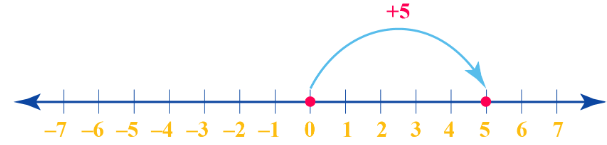The next number in the given problem is -10, which is negative. We move 10 units to the left side from 5.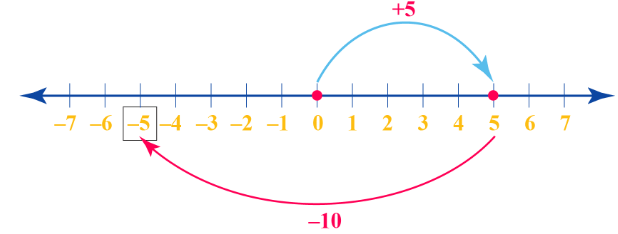Finally, we reach at -5. Therefore, the value of 5 + (-10) = -5

Subtraction of Integers

Subtracting integers is the process of finding the difference between two or more integers where the final value might increase or decrease depending on the integer being positive or negative. The different rules and the possible cases for the subtraction of integers are given in the following section.

Rules of Integers in Subtraction

In order to carry out the subtraction of two integers, we use the following rules:

• Convert the operation into an addition problem by changing the sign of the subtrahend.
• Apply the same rules of addition of integers and solve the problem thus obtained in the above step.

Example: Subtract the given integers: 7 - 10
Solution: 7 - 10 can be written as (+ 7) - (+)10

• Convert the given expression into an addition problem and change the sign of the subtrahend, so, we get: 7 + (-10)
• Now, the rules for this operation will be the same as for the addition of two integers.
• Here, the absolute values of 7 and (-10) are 7 and 10 respectively.
• Their difference (larger number - smaller number) is 10 - 7 = 3.
• Now, among 7 and 10, 10 is the larger number and its original sign “-”.
• Hence, the result gets a negative sign, "-”.
• Therefore, 7 - 10 = -3

Multiplication of Integers

For the multiplication of integers, we use the following rules given in the table. The different rules and the possible cases for the multiplication of integers are given in the following section.

Rules of Integers in Multiplication
In order to carry out the multiplication of two integers, we use the following rules: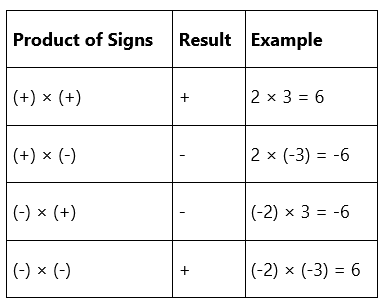Example: Multiply (-6) × 3
Solution: Using the rules of multiplication of integers, when we multiply a positive and negative integer, the product has a negative sign.
Therefore, (-6) × 3 = -18

Division of Integers

Division of integers means equal grouping or dividing an integer into a specific number of groups. For the division of integers, we use the rules given in the following table. The different rules and the possible cases for the division of integers are given in the following section

Rules of Integers in Division

In order to carry out the division of two integers, we use the following rules.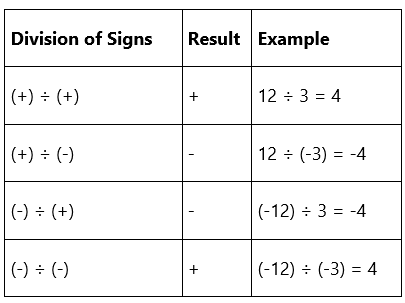Example: Divide (-15) ÷ 3
Solution: Using the rules of division of integers, when we divide a negative integer by a positive integer, the quotient has a negative sign.
Therefore, (-15) ÷ 3 = -5

Properties of Integers

The main properties of Integers are as follows:

1. Closure Property The closure property states that the set is closed for any particular mathematical operation. Z is closed under addition, subtraction, multiplication, and division of integers. For any two integers, a and b:

• a + b ∈ Z
• a - b ∈ Z
• a × b ∈ Z
• a/b ∈ Z

2. Associative Property

According to the associative property, changing the grouping of two integers does not change the result of the operation. The associative property applies to the addition and multiplication of two integers.
For any two integers, a and b:

• a + (b + c) = (a + b) + c
• a × (b × c) = (a × b) × c

3. Commutative Property
According to the commutative property, changing the position of the operands in an operation does not affect the result. The addition and multiplication of integers follow the commutative property.

For any two integers, a and b:

• a + b = b + a
• a × b = b × a

4. Distributive Property
The distributive property states that for any expression of the form a (b + c), which means a × (b + c), operand a can be distributed among the operands b and c as: (a × b) + (a × c) that is,
a × (b + c) = (a × b) + (a × c)

The additive inverse property states that the addition operation between any integer and its negative value will give the result as zero (0).
For any integer, a:
a + (-a) = 0

6. Multiplicative Inverse Property
The multiplicative inverse property states that the multiplication operation between any integer and its reciprocal will give the result as one (1).
For any integer, a: a × 1/a = 1

7. Identity Property
Integers follow the identity property for addition and multiplication operations. The additive identity property states that when zero is added to an integer, it results in the integer itself. This means, a + 0 = a
Similarly, the multiplicative identity states that when 1 is multiplied to any integer, it results in the integer itself. This means, a × 1 = a

Integers Examples

Example 1: Can you identify the property of the integer used in the following expression? 3 + (7 + 2) = (3 + 7) + 2
Solution:
The given expression, 3 + (7 + 2) = (3 + 7) + 2, shows the associative property of integers, which says that changing the grouping of two integers does not change the result of the operation because 3 + (7 + 2) = (3 + 7) + 2 = 12.

Example 2: Add the following integers: (-9) + (-5)
Solution:
According to the rules of integers in addition, when both the integers have the same signs, we add the absolute values of integers and give the same sign as that of the given integers to the result.
The absolute values of the given integers is 9 and 5.
So we will add 9 + 5 = 14 and the sign of the sum will be negative.
Therefore, (-9) + (-5) = -14

The document Integers - Notes | Study Mathematics for ACT - ACT is a part of the ACT Course Mathematics for ACT.
All you need of ACT at this link: ACT

## Mathematics for ACT

19 videos|9 docs|3 tests

## Mathematics for ACT

19 videos|9 docs|3 tests

Track your progress, build streaks, highlight & save important lessons and more!

,

,

,

,

,

,

,

,

,

,

,

,

,

,

,

,

,

,

,

,

,

;